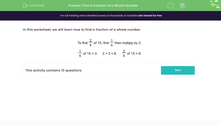# Find a Fraction of a Whole Number

In this worksheet, students evaluate a fraction of a whole number.Key stage:  KS 2

Curriculum topic:   Number: Multiplication and Division

Curriculum subtopic:   Solve Multiplication/Division Problems with Fractions

Difficulty level:#### Worksheet Overview

In this activity, we'll look at how to find a fraction of a whole number.If this sounds, complicated... don't panic!  We'll go through the steps below and you'll see it's easier than it sounds.

STEP 1To do this, we divide 15 by our denominator.

(Remember, this is the number that is on the bottom or down below our fraction line)

In this case, our denominator is so we need to do the following division calculation:

15 ÷ 5 = 3

STEP 2

Let's look again at the original question.If you said that we should multiply our answer by 2, you'd be correct!

3 × 2 = 6

There we go, the correct answer is 6.

Always remember to start off by working out what 1/5 is first, and then you can work the rest out from there!

Let's give this a go.

### What is EdPlace?

We're your National Curriculum aligned online education content provider helping each child succeed in English, maths and science from year 1 to GCSE. With an EdPlace account you’ll be able to track and measure progress, helping each child achieve their best. We build confidence and attainment by personalising each child’s learning at a level that suits them.

Get started••••